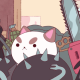# Ticha Sethapakdi

## 01 Feb 2014Soooo unfortunately my lenticular print turned out kinda NOT AT ALL the way I wanted it to. :(  Which prompted me to make another one! I actually like this one more than my old one, so I guess the print was a disaster for a good cause.

In the interest of allocating enough time to do the things for Project 2, I decided to go with something simple for my second GIF. I found it really funny how we all had to bounce up and down to view each other’s prints, so I decided to create an animation that mimicked that motion.Hopefully it’ll work this time…..

```1 2 3 4 5 6 7 8 9 10 11 12 13 14 15 16 17 18 19 20 21 22 23 24   //jigglycube   num = 6; gap = 30; growthj = 2; growthi = 1.5; growthh = 1.5;   //fake background fill translate([-400,300,0]) {rotate([0,90,310]) color("black") {cube([2500,2500,1],center=true);}}   //height for(h = [0 : num]) { //rows for(i = [0 : num] ) { //columns for(j = [0 : num]) { color([0.6+4*sin(20*h)/2, 0.6+cos(24*j)/3, 0.6+cos(15*(i+j+1))/4]) { translate([-gap*j + j*4.5*cos(\$t*360)*growthj , -gap*i + i*5*cos(\$t*360)*growthi, gap*h + h*5*sin(\$t*360)*growthh]) cube(size=gap, center=true); }} }}```
1.Golan Levin